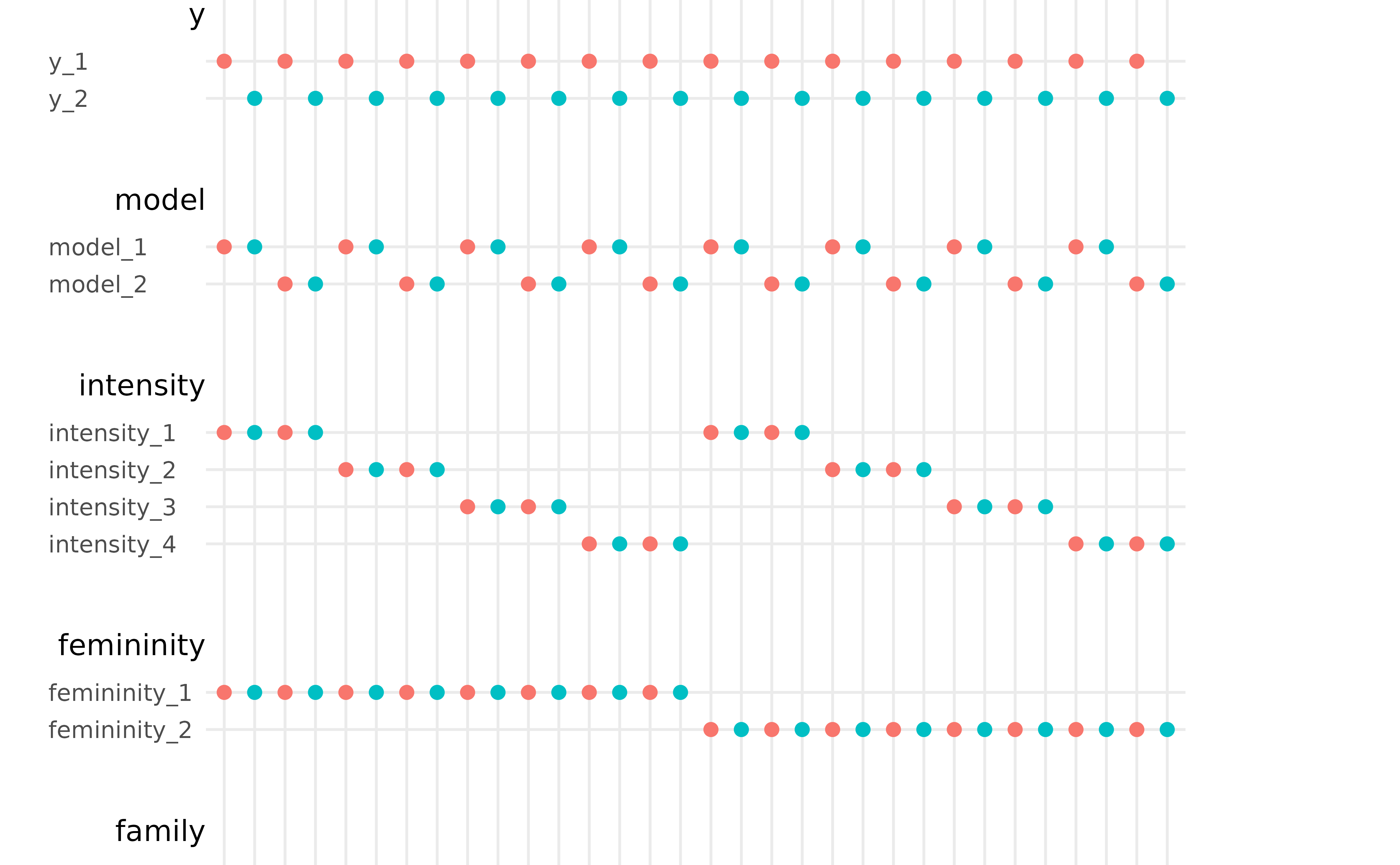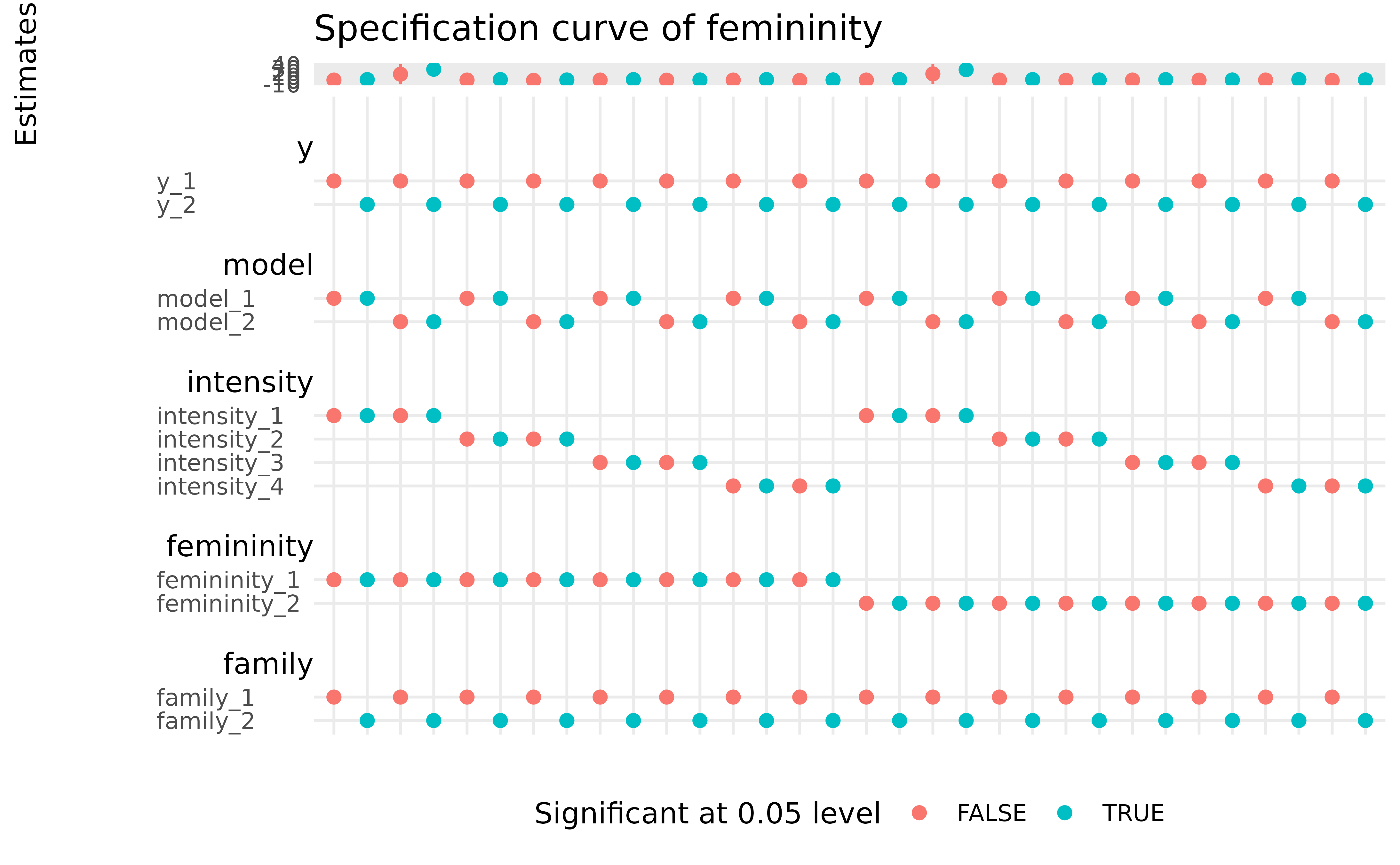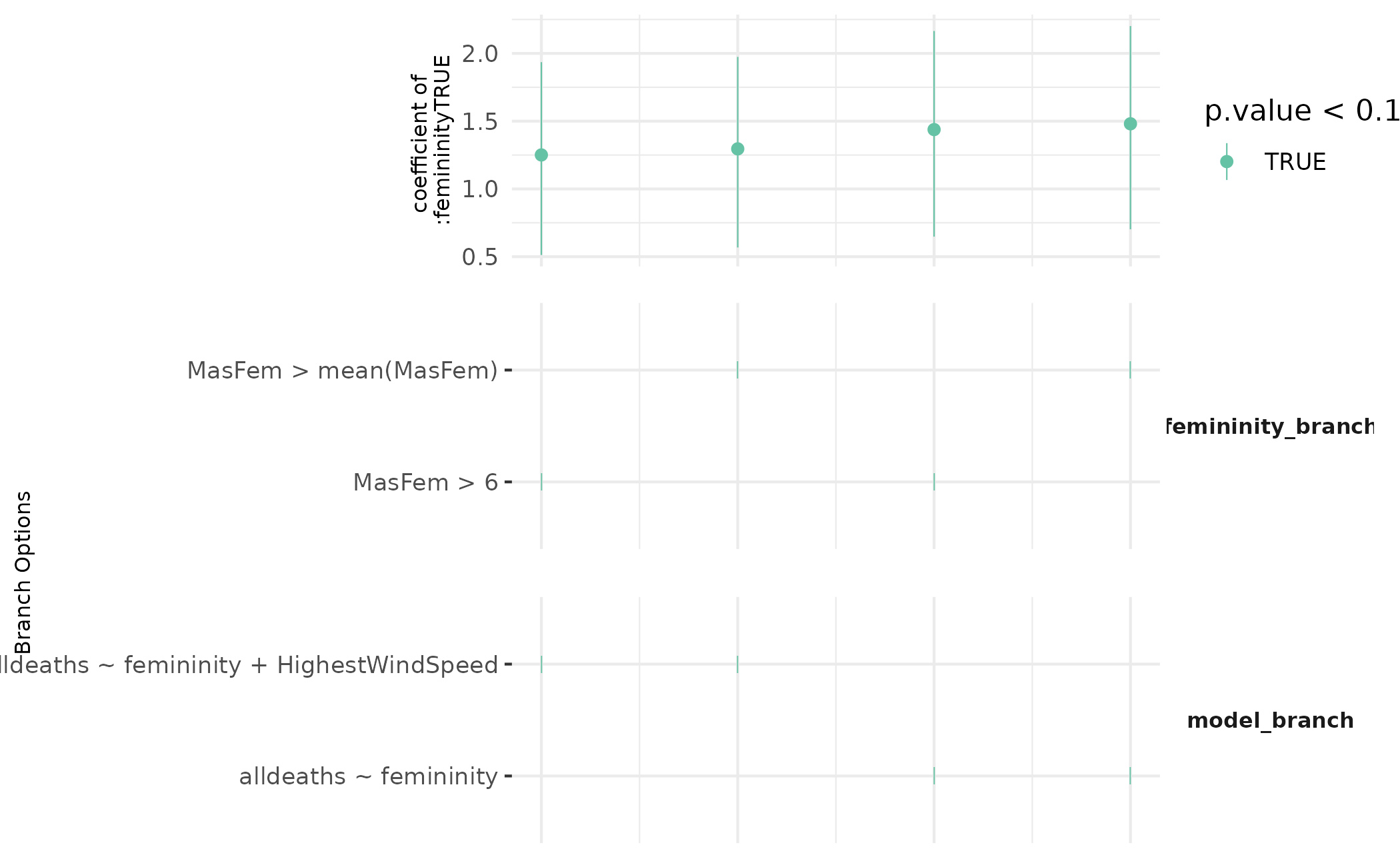spec_curve returns the specification curve as proposed by Simonsohn, Simmons, and Nelson (2020) <doi:10.1038/s41562-020-0912-z>. spec_curve are available for mverse objects fitted with lm_mverse(), glm_mverse(), and glm.nb_mverse(). Notice that the order of universes may not correspond to the order in the summary table.

spec_curve(.object, var, ...)

# S3 method for lm_mverse
spec_curve(
.object,
var,
conf.int = TRUE,
conf.level = 0.95,
option = names(multiverse::parameters(.object)),
universe_order = FALSE,
color_order = FALSE,
color = NULL,
branch_order = NULL,
point_size = 0.25,
grid_size = 2,
point_alpha = 1,
brewer_palette = "Set2",
yaxis_text_size = 8,
...
)

# S3 method for glm_mverse
spec_curve(
.object,
var,
conf.int = TRUE,
conf.level = 0.95,
option = names(multiverse::parameters(.object)),
universe_order = FALSE,
color_order = FALSE,
color = NULL,
branch_order = NULL,
point_size = 0.25,
grid_size = 2,
point_alpha = 1,
brewer_palette = "Set2",
yaxis_text_size = 8,
...
)

# S3 method for glm.nb_mverse
spec_curve(
.object,
var,
conf.int = TRUE,
conf.level = 0.95,
option = names(multiverse::parameters(.object)),
universe_order = FALSE,
color_order = FALSE,
color = NULL,
branch_order = NULL,
point_size = 0.25,
grid_size = 2,
point_alpha = 1,
brewer_palette = "Set2",
yaxis_text_size = 8,
...
)

## Source

Uri Simonsohn, Joseph P. Simmons, and Leif D. Nelson. (2020). “Specification curve analysis” Nature Human Behaviour, 4, 1208–14. doi:10.1038/s41562-020-0912-z

## Arguments

.object

a glm.nb_mverse object.

var

name for the variable to show.

...

ignored.

conf.int

when TRUE (default), the estimate output includes the confidence intervals.

conf.level

the confidence level of the confidence interval returned using conf.int = TRUE. Default value is 0.95.

option

a vector of branches to show the options included.

universe_order

when TRUE, order the universes according to the order in the summary table.

color_order

when TRUE, the estimated value will be ordered according to the color.

color

an expression to indicate how colors are assigned to markers. By default, colors are assigned based on 'p.value <= 0.05'.

branch_order

name for the branch to order.

point_size

size of points on the top plot.

grid_size

size of points on the bottom plot.

point_alpha

alpha level of points and point ranges.

brewer_palette

name of colorbrewer palette for the plot.

yaxis_text_size

text size of y-axis label

## Value

a specification curve plot for the estimates

## Examples

# \donttest{

# Display a specification curve for \code{lm} models
# fitted across the multiverse.
femininity <- mutate_branch(
MasFem > 6, MasFem > mean(MasFem)
)
model <- formula_branch(
alldeaths ~ femininity,
alldeaths ~ femininity + HighestWindSpeed
)
mv <- mverse(hurricane) %>%
lm_mverse()
spec_curve(mv, var = "femininityTRUE")# plot based on 90% confidence interval
spec_curve(mv, var = "femininityTRUE", color = p.value < .1)# }
# \donttest{

# Display a specification curve for \code{glm} models
# fitted across the multiverse.
femininity <- mutate_branch(
MasFem > 6, MasFem > mean(MasFem)
)
model <- formula_branch(
alldeaths ~ femininity,
alldeaths ~ femininity + HighestWindSpeed
)
fam <- family_branch(gaussian)
mv <- mverse(hurricane) %>%
glm_mverse()
spec_curve(mv, var = "femininityTRUE")# plot based on 90% confidence interval
spec_curve(mv, var = "femininityTRUE", color = p.value < .1)# }
# \donttest{

# Display a specification curve for \code{glm.nb} models
# fitted across the multiverse.
femininity <- mutate_branch(
MasFem > 6, MasFem > mean(MasFem)
)
model <- formula_branch(
alldeaths ~ femininity,
alldeaths ~ femininity + HighestWindSpeed
)
mv <- mverse(hurricane) %>%